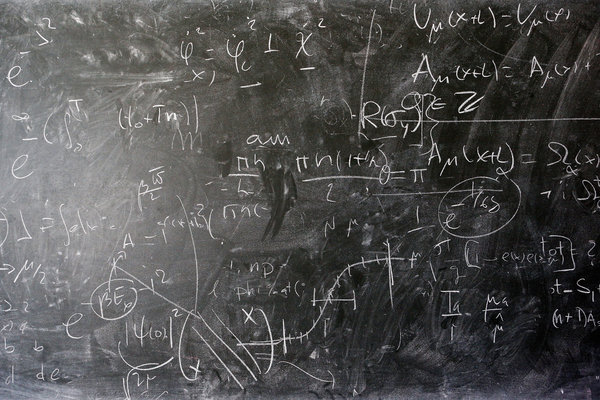## Stupid math tricks on social mediaRecently as documented by this New York Times article, this rather ambiguous math problem (middle-school pre-algebra level) has been making the rounds. The article references the Yanny/Laurel controversy as well as the dress that people saw as different colors. I didn’t really take a side in either of those, but this one highlights some of the potentially ambiguous nature of mathematical notation.

The math problem in question:

`x = 8 ÷ 2(2+2)`

First, let me state the obvious: this is poorly written, and normally when using algebraic multiplication notation, i.e. putting the terms right next to each other, one would also use the fraction bar or vinculum instead of the obelus (“÷”) symbol. So instead, one would write either the equivalent of:

`x = (8÷2)(2+2)`

or

`x = 8 ÷ (2(2+2))`

Indeed, in the computer programming language Forth, which I have spent a bit of time dabbling in (still need to package up some of the code I’ve written into a proper release), there is no need to memorize the time-honored mnemonic of Please Excuse My Dear Aunt Sally for the order of operations (parentheses, then exponentiation, then multiplication and division, then addition and subtraction). One would code either of the following:

`8 2 / 2 2 + *`

or

`8 2 2 + 2 * /`

The first one gives you 16 as in the first example above (with the 8÷2 grouped together), the second one gives you 1 as in in the second example above (with the 8 being a term by itself).

The article’s author seems to imply the “correct” answer is 16. The fact that there’s any debate on the correct answer, and that I apparently arrived at the “wrong” answer despite my stellar math background (I aced every high school math class except geometry and that one only because I blew off all the major projects), says to me there’s something wrong with how the question is written. Nobody, and I mean nobody, uses this half-baked obelus symbol notation for division at any serious level of mathematics unless they are printing calculator buttons. This type of problem is one reason. The other is that a sloppily written (or blurry-printed!) obelus can look way too much like an addition symbol. Indeed, the author of the article goes on to state:

No professional mathematician would ever write something so obviously ambiguous. We would insert parentheses to indicate our meaning and to signal whether the division should be carried out first, or the multiplication.

The lessons to be learned here are:

1. Be careful how you write math problems; and
2. Apparently anything is fair game to debate on social media.